### IMO Shortlist 2008 problem C4

Kvaliteta:
Avg: 0,0
Težina:
Avg: 7,0
Let$n$ and$k$ be positive integers with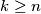$k \geq n$ and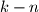$k - n$ an even number. Let$2n$ lamps labelled$1$,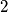$2$, ...,$2n$ be given, each of which can be either on or off. Initially all the lamps are off. We consider sequences of steps: at each step one of the lamps is switched (from on to off or from off to on).

Let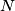$N$ be the number of such sequences consisting of$k$ steps and resulting in the state where lamps$1$ through$n$ are all on, and lamps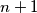$n + 1$ through$2n$ are all off.

Let$M$ be number of such sequences consisting of$k$ steps, resulting in the state where lamps$1$ through$n$ are all on, and lamps$n + 1$ through$2n$ are all off, but where none of the lamps$n + 1$ through$2n$ is ever switched on.

Determine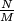$\frac {N}{M}$.

Author: Bruno Le Floch and Ilia Smilga, France
Izvor: Međunarodna matematička olimpijada, shortlist 2008